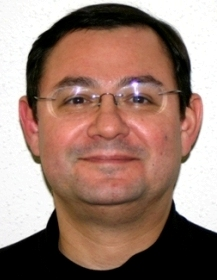Select PageSpeaker: Michael Mascagni Date: Oct 27, 2023, 2:15 – 3:10 PM Abstract: We are interested in using stochastic (Monte Carlo) methods to solve problems that are relevant to electrostatics and specifically to semiconductor design. Thus, we begin by introducing what Monte Carlo are and show how they can be used to compute several numerical quantities. We then focus on Monte Carlo methods using the Walk on Spheres technique to solve partial differential equations of the elliptic type. These are equations that describe a state of physical equilibrium such as we see in electrostatics. One such important problem in electrostatics is computing the capacitance of an object in 3D. We discuss how this is done using both conventional deterministic methods and the Walk on Spheres technique. We then show how one can use similar ideas from stochastic computing to compute relative capacitance and then mutual capacitance. Mutual capacitance is an important quantity used to evaluate the electrostatic suitability of semiconductor designs. As such, fast and accurate methods for mutual capacitance computations are important in facilitating the semiconductor design process. We then consider the computation of capacitance as a computational paradigm. Using the Walk on Spheres method is a very efficient computational approach for capacitance extraction, and so it has become the method of choice by a variety of vendors of software for semiconductor design and in many aspects of computer graphics. In particular, the Walk on Spheres technique has significantly improved the performance of the ZENO code developed at NIST by the author and various collaborators. At present, the author is seeking funding to continue this work using CHIPS funding. Links and comments: This talk will be virtual only, https://fsu.zoom.us/my/mascagni